ROCKET THERMODYNAMICS

A thorough understanding of thermodynamics is not a necessary requirement for the study of rocketry. As long as the temperature, molecular weight, of specific heat ratio of the exhaust products is known, the rocket equations can be solved. It is, however, often useful to be able to derive these quantities for one's self. In this article we provide an overview of the chemical thermodynamics applicable to rocket propulsion. It is assumed the reader has at least an elementary understanding of thermodynamics, as it is not our intent to fully explain the fundamentals.

Because of the complexity and tedious nature of the calculations, an easier method has been provided to readers of this web site. A series of propellant combustion charts are available from which one can obtain optimum mixture ratio, adiabatic flame temperature, gas molecular weight, and specific heat ratio for some common rocket propellants. These charts were developed using the methods described in this article.

Enthalpy

Enthalpy is a measure of the total energy of a thermodynamic system. It includes the internal energy, which is the energy required to create a system, and the amount of energy required to make room for it by displacing its environment and establishing its volume and pressure. The unit of measurement for enthalpy in the International System of Units (SI) is the joule, but other historical, conventional units are still in use, such as the BTU and the calorie.

The enthalpy is the preferred expression of system energy changes in many chemical, biological, and physical measurements, because it simplifies certain descriptions of energy transfer. This is because a change in enthalpy takes account of energy transferred to the environment through the expansion of the system under study.

The total enthalpy, H, of a system cannot be measured directly. Thus, change in enthalpy, ΔH, is a more useful quantity than its absolute value. The change ΔH is positive in endothermic reactions, and negative in heat-releasing exothermic processes. ΔH of a system is equal to the sum of non-mechanical work done on it and the heat supplied to it.

For quasistatic processes under constant pressure, ΔH is equal to the change in the internal energy of the system, plus the work that the system has done on its surroundings. This means that the change in enthalpy under such conditions is the heat absorbed (or released) by a chemical reaction. Enthalpies for chemical substances at constant pressure assume standard state: most commonly 1 bar (0.1 MPa) pressure. Standard state does not, strictly speaking, specify a temperature, but expressions for enthalpy generally reference the standard enthalpy of formation at 25oC (298.15 K).

While H denotes the total enthalpy of a system, h is specific enthalpy, i.e. the enthalpy per mole or unit mass of a substance. We will simply refer to both as enthalpy, since the context will make it clear which is applicable.

The enthalpy of formation, or heat or formation, hof , is the enthalpy of a substance at the base conditions of 25oC and 0.1 MPa pressure. The enthalpy in any other state, relative to this base, would be found by adding the change of enthalpy between 25oC and 0.1 MPa and the given state to the enthalpy of formation. That is, the enthalpy at any temperature and pressure, hT,P, is

hT,P = (hof )298,0.1 MPa + (Δh)298,0.1 MPa --> T,P

where the term (Δh)298,0.1 MPa --> T,P represents the difference in enthalpy between any given state and the enthalpy at 298.15 K, 0.1 MPa. For convenience we usually drop the subscripts in the examples that follow.

In most cases the substances that comprise the reactants and products in a chemical reaction are not at a temperature of 25oC and a pressure of 0.1 MPa. Therefore the change of enthalpy between 25oC and 0.1 MPa and the given state must be known. In the case of a solid or liquid, this change of enthalpy can usually be found from a table of thermodynamic properties or from specific heat data. In the case of gases, this change of enthalpy can be found as follows:

Assume ideal gas behavior between 25oC, 0.1 MPa, and the given state. In this case, the enthalpy is a function of the temperature only, and can be found by use of an equation, from tabulated values of enthalpy as a function of temperature (which assumes ideal gas behavior), or calculated from specific heat capacity, Cpo, where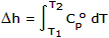NIST Chemistry WebBook provides equations for (ho-ho298) and Cpo for most substances, and tables can be generated using the web site NIST-JANAF Termochemical Tables. (ho-ho298) is Δh, where ho298 refers to 25oC or 298.15 K. The superscript o is used to designate that this is the enthalpy at 0.1 MPa pressure, based on ideal gas behavior, that is, the standard-state enthalpy.

When the deviation from ideal gas behavior is significant, other procedures must be employed, but that won't be necessary in our case.

Consider a given combustion process that takes place adiabatically and with no work or changes in kinetic or potential energy involved. For such a process the temperature of the products is referred to as the adiabatic flame temperature. With the assumptions of no work and no changes in kinetic or potential energy, this is the maximum temperature that can be achieved for the given reactants because any heat transfer from the reacting substances and any incomplete combustion would tend to lower the temperature of the products. For a given fuel and given pressure and temperature of the reactants, the maximum adiabatic temperature that can be achieved is with a stoichiometric mixture.

The following example shows how the adiabatic flame temperature may be found. The dissociation that takes place in the combustion products, which has a significant effect on the adiabatic flame temperature, will be considered in the next section.

Suppose we react a stoichiometric mixture of gaseous methane and gaseous oxygen at an initial temperature of 25oC and 4 MPa pressure. Our reaction equation is

CH4 + 2 O2CO2 + 2 H2O

We assume ideal gas behavior for all the constituents. In this case the first law of thermodynamics reduces to

HR = HP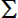R ni[hof + Δh]i =P ne[hof + Δh]e

where HR is the enthalpy of the reactants, HP the enthalpy of the products, hof the enthalpy of formation of each constituent, Δhi the change in enthalpy of each constituent in the reactants at the initial temperature, and Δhe the change in enthalpy of each constituent in the products at the adiabatic flame temperature.

Below is a table giving enthalpy of formation and ideal gas enthalpy for several of the products that will result from a methane/oxygen reaction. Note that in the simplified reaction considered above, we have only CO2 and H2O on the right side of the equation. The additional substances will appear when we consider dissociation in the next section.

 TemperatureK MethaneCH4(3/31/61) Carbon DioxideCO2(9/30/65) Carbon MonoxideCO(9/30/65) Oxygen, DiatomicO2(9/30/65) Water (gas)H2O(3/31/61) Hydrogen, DiatomicH2(3/31/61) HydroxylOH(3/31/66) (hfo)298 = -74.873M = 16.043 (hfo)298 = -393.522M = 44.010 (hfo)298 = -110.529M = 28.010 (hfo)298 = 0M = 31.999 (hfo)298 = -241.827M = 18.015 (hfo)298 = 0M = 2.0159 (hfo)298 = 39.463M = 17.007 (ho-ho298)kJ/mol (ho-ho298)kJ/mol (ho-ho298)kJ/mol (ho-ho298)kJ/mol (ho-ho298)kJ/mol (ho-ho298)kJ/mol (ho-ho298)kJ/mol 0 -10.024 -9.364 -8.669 -8.682 -9.904 -8.468 -9.171 100 -6.698 -6.456 -5.770 -5.778 -6.615 -5.293 -6.138 200 -3.368 -3.414 -2.858 -2.866 -3.280 -2.770 -2.975 298 0.000 0.000 0.000 0.000 0.000 0.000 0.000 300 0.066 0.067 0.054 0.054 0.063 0.054 0.054 400 3.861 4.008 2.975 3.029 3.452 2.958 3.033 500 8.200 8.314 5.929 6.088 6.920 5.883 5.991 600 13.130 12.916 8.941 9.247 10.498 8.812 8.941 700 18.635 17.761 12.021 12.502 14.184 11.749 11.903 800 24.675 22.815 15.175 15.841 17.991 14.703 14.878 900 31.205 28.041 18.397 19.246 21.924 17.682 17.887 1000 38.179 33.405 21.686 22.707 25.978 20.686 20.933 1100 45.549 38.894 25.033 26.217 30.167 23.723 24.025 1200 53.270 44.484 28.426 29.765 34.476 26.794 27.158 1300 61.302 50.158 31.865 33.351 38.903 29.907 30.342 1400 69.608 55.907 35.338 36.966 43.447 33.062 33.568 1500 78.153 61.714 38.848 40.610 48.095 36.267 36.840 1600 86.910 67.580 42.384 44.279 52.844 39.522 40.150 1700 95.853 73.492 45.940 47.970 57.685 42.815 43.501 1800 104.960 79.442 49.522 51.689 62.609 46.150 46.890 1900 114.212 85.429 53.124 55.434 67.613 49.522 50.308 2000 123.592 91.450 56.739 59.199 72.689 52.932 53.760 2100 133.087 97.500 60.375 62.986 77.831 56.379 57.241 2200 142.684 103.575 64.019 66.802 83.036 59.860 60.752 2300 152.371 109.671 67.676 70.634 88.295 63.371 64.283 2400 162.141 115.788 71.346 74.492 93.604 66.915 67.839 2500 171.984 121.926 75.023 78.375 98.964 70.492 71.417 2600 181.893 128.085 78.714 82.274 104.370 74.090 75.015 2700 191.862 134.256 82.408 86.199 109.813 77.718 78.634 2800 201.885 140.444 86.115 90.144 115.294 81.370 82.266 2900 211.958 146.645 89.826 94.111 120.813 85.044 85.918 3000 222.076 152.862 93.542 98.098 126.361 88.743 89.584 3200 242.431 165.331 100.998 106.127 137.553 96.199 96.960 3400 262.925 177.849 108.479 114.232 148.854 103.738 104.387 3600 283.536 190.405 115.976 122.399 160.247 111.361 111.859 3800 304.248 202.999 123.495 130.629 171.724 119.064 119.378 4000 325.045 215.635 131.026 138.913 183.280 126.846 126.934 4200 345.918 228.304 138.578 147.248 194.903 134.700 134.528 4400 366.855 241.003 146.147 155.628 206.585 142.624 142.156 4600 387.849 253.734 153.724 164.046 218.325 150.620 149.816 4800 408.893 266.500 161.322 172.502 230.120 158.682 157.502 5000 429.982 279.295 168.929 180.987 241.957 166.808 165.222 5200 451.110 292.123 176.548 189.502 253.839 174.996 172.967 5400 472.274 304.984 184.184 198.037 265.768 183.247 180.736 5600 493.469 317.884 191.832 206.593 277.738 191.556 188.531 5800 514.692 330.821 199.489 215.166 289.746 199.924 196.351 6000 535.942 343.791 207.162 223.756 301.796 208.346 204.192 The thermochemical data are calculated from the JANAF Thermochemical Tables,Thermal Research Laboratory, The Dow Chemical Company, Midland, Michigan.The date each table was issued is indicated.

Knowing the temperature of the reactants, we can easily calculate HR. Since the reactant temperature in our example is equal to the base temperature of 25oC, Δhi = 0 and we need only to sum the enthalpies of formation of the reactants. From the table above we see that for diatomic oxygen (hfo)298 = 0, which is typical for diatomic gases, and for methane we find (hfo)298 = -74.873 kJ/mol. Therefore, we have

HR =i  ni [hof + Δh]i = 1 × [-74.873 + 0] + 2 × [0 + 0] = -74.873 kJ

For the products, we have

HP =e  ne [hof + Δh]e = 1 × [-393.522 + ΔhCO2] + 2 × [-241.827 + ΔhH2O] = -74.873 kJ

By trial-and-error solution, a temperature of the products is found that satisfies this equation. Suppose we've already performed several trials and have begun to hone in on a solution. For the next trial, assume TP = 5,400 K.

HP = 1 × [-393.522 + 304.984] + 2 × [-241.827 + 265.768] = -40.656 kJ

Since HP > HR, our assumed temperature is too high. Let's now try TP = 5,200 K.

HP = 1 × [-393.522 + 292.123] + 2 × [-241.827 + 253.839] = -77.375 kJ

We can now interpolate to find the adiabatic flame temperature.

TP = (-74.873 – (-77.375)) / (-40.656 – (-77.375)) × (5,400 – 5,200) + 5,200 = 5,214 K

Cryogenic Reactants

From the preceding example it can be seen that the initial temperature of the reactants has an effect on the final temperature of the products. For storable propellants, temperature variations within the normal liquid range have only a small effect on engine performance. For hypergols, a 10oC change in reactant temperature results in about 0.1% change in specific impulse. We can, therefore, safely assume that the initial temperature of storable propellants is the standard enthalpy of formation temperature of 25oC. This assumption has the added benefit of simplifying the calculations as we need only look up the enthalpies of formations for the reactants without making any further adjustments for temperature.

Cryogenic propellants are another story, as their extremely low temperatures have a significant effect on performance. We must further take into consideration that the readily available thermochemical data for these substances is gaseous state rather than liquid. An adjustment must be made to account for the change of state.

Let's consider the example of cryogenic oxygen, which has a boiling point of -183oC (90 K). We start with gaseous oxygen at 298 K, which has an enthalpy of formation of 0 kJ/mol. We then add the change in enthalpy required to lower the temperature from 298 K to 90 K. From the enthalpy tables we estimate that this adjustment is -6.06 kJ/mol. Next we deduct the enthalpy of vaporization to account for the change of state from gas to liquid. The enthalpy of vaporization of oxygen is 6.82 kJ/mol. Therefore, the enthalpy of liquid oxygen reactant is

ho(liquid) = hof + Δh298 -> 90 – Δhvap = 0 – 6.06 – 6.82 = -12.88 kJ/mol

We now turn our attention to chemical equilibrium. Here we will consider a chemical reaction involving only one phase, which is referred to as a homogeneous chemical reaction. In our case we will be considering a gaseous phase, but the basic considerations apply to any phase.

Consider a vessel that contains four compounds, A, B, C, and D, which are in chemical equilibrium at a given pressure and temperature. Let the number of moles of each component be designated nA, nB, nC, and nD. Further, let the chemical reaction that takes place between these four constituents be

vAA + vBB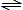vCC + vDD

where the v's are the stoichiometric coefficients. It should be emphasized that there is a very definite relation between the v's (the stoichiometric coefficients) whereas the n's (the number of moles present) for any constituent can be varied simply by varying the amount of that component in the reaction vessel.

To determine the equilibrium composition for a chemical reaction, we must be able to determine the activity of the various constituents in the mixture. We do so by applying the concept of the equilibrium constant, K. Assuming the ideal gas model, which will be appropriate for our examples and applications, the chemical equilibrium equation can be written as

K = (yCVC × yDVD) / (yAVA × yBVB) × (P/Po)VC+VD-VA-VB

where y is the equilibrium mole fraction of the A, B, C and D constituents, v is the stoichiometric coefficient for each constituent, and the term P/Po represents the ratio of pressure at which the reaction occurs to the standard state pressure.

The equation is written in this form because it demonstrates quite clearly the influence of various factors on the equilibrium composition (the y's). That is, we know that temperature and pressure both influence the equilibrium composition. It can be seen that the influence of temperature enters through the value of K (which is a function of temperature only) and the influence of pressure though the term (P/Po)VC+VD-VA-VB.

It should be noted that the equilibrium of combustion gases is very sensitive to temperature. Products existing at a high combustion temperature are very different from those existing at a lower combustion temperature. At high temperatures, dissociation of the products occurs, as the thermal energy causes the break up of molecules into simpler and monatomic constituents. Dissociation reactions are reversible (as indicated by the double arrow in the equations), so as the gases cool, recombination can occur.

Let's now revisit our previous example and employ a procedure for determing the equilibrium composition of the combustion products.

It is convenient to view the over-all process as though it occurred in two separate steps, a combustion process followed by a heating and dissociation of the combustion products. The combustion reaction is

CH4 + 2 O2CO2 + 2 H2O

There are several dissociation reactions that will come into play, but we'll consider the two most dominant in this particular example.

CO2CO + 1/2 O2
H2O1/2 H2 + OH

That is, the energy released by the combustion of CH4 and O2 heats the CO2 and H2O to high temperature, resulting in the dissociation of part of the CO2 to CO and O2, and part of the H2O to H2 and OH. Thus, the overall reaction can be written

CH4 + 2 O2a CO2 + b CO + c O2 + d H2O + e H2 + f OH

where the unknown coefficients a, b, c, d, e, and f must be found by solution of the equilibrium equations associated with the dissociation reactions.

From the combustion reaction we find that the initial compositions for the dissociation reactions are 1 mole CO2 and 2 moles H2O. Therefore, letting z' be the number of moles of CO2 dissociated, and z" be the number of moles of H2O dissociated, we find

```
CO2CO   +   1/2 O2

Initial:              1        0        0
Change:               -z'      +z'      +z'/2
_____________________________________________

At equilibrium:     (1-z')     z'       z'/2

H2O1/2 H2  +  OH

Initial:              2        0         0
Change:               -z"      +z"/2     +z"
_____________________________________________

At equilibrium:     (2-z")     z"/2      z"

```

Therefore the overall reaction is

CH4 + 2 O2(1-z') CO2 + z' CO + z'/2 O2 + (2-z") H2O + z"/2 H2 + z" OH

And the total number of moles at equilibrium for each reaction is

n' = (1-z') + z' + z'/2 = 1 + z'/2

n" = (2-z") + z"/2 + z" = 2 + z"/2

The equilibrium mole fractions are

yCO2 = (1-z')/(1+z'/2)
yCO = z'/(1+z'/2)
yO2 = (z'/2)/(1+z'/2)

yH2O = (2-z")/(2+z"/2)
yH2 = (z"/2)/(2+z"/2)
yOH = z"/(2+z"/2)

Substituting these quantities along with P = 4 MPa, we have the equilibrium equations.

K' = yCO yO21/2 / yCO2 × (P/Po)1+1/2-1 = [z'/(1+z'/2)] × [(z'/2)/(1+z'/2)]1/2 / [(1-z')/(1+z'/2)] × (4/0.1)1/2

K" = yH2 yOH2 / yH2O2 × (P/Po)1+2-2 = [(z"/2)/(2+z"/2)] × [z"/(2+z"/2)]2 / [(2-z")/(2+z"/2)]2 × (4/0.1)1

Values of the equilibrium constants are found in the following table:

 Logarithms to the Base e of the Equilibrium Constant K TemperatureK CO2 = CO + 1/2 O2 H2O = 1/2 H2 + OH 298 -103.762 -106.208 500 -57.616 -60.281 1000 -23.529 -26.034 1200 -17.871 -20.283 1400 -13.842 -16.099 1600 -10.830 -13.066 1800 -8.497 -10.657 2000 -6.635 -8.728 2200 -5.120 -7.148 2400 -3.860 -5.832 2600 -2.801 -4.719 2800 -1.894 -3.763 3000 -1.111 -2.937 3200 -0.429 -2.212 3400 0.169 -1.576 3600 0.701 -1.009 3800 1.176 -0.501 4000 1.599 -0.044 4500 2.490 0.920 5000 3.197 1.689 5500 3.771 2.318 6000 4.245 2.843

By trial-and-error solution, a temperature of the products must be found that satisfies the equations. The steps are

• Select a trial value for TP.
• Obtain ln(K) values from the table and calculate values of K.
• By trial-and-error, calculate the values of z.
• Calculate the number of moles of each product.
• Calculate the total enthalpy of the products.
• Compare the product enthalpy to the reactant enthalpy.
• If HP ≠ HR, select a new trial value of TP and repeat.
• When close, interpolate to find final TP.

Let's assume TP = 3,600 K, we have

ln(K') = 0.701,   K' = 2.016,   z' = 0.4309

ln(K") = -1.009,   K" = 0.3646,   z" = 0.4587

CH4 + 2 O20.5691 CO2 + 0.4309 CO + 0.2154 O2 + 1.5413 H2O + 0.2294 H2 + 0.4587 OH

HP = -117.66 kJ/mol, which is < HR

Assume TP = 3,800 K and repeat.

ln(K') = 1.176,   K' = 3.241,   z' = 0.5285

ln(K") = -0.501,   K" = 0.6059,   z" = 0.5294

CH4 + 2 O20.4715 CO2 + 0.5285 CO + 0.2643 O2 + 1.4706 H2O + 0.2647 H2 + 0.5294 OH

HP = -35.94 kJ/mol, which is > HR

Calculate adiabatic flame temperature by interpolation.

TP = (-74.873 – (-117.66)) / (-35.94 – (-117.66)) × (3,800 – 3,600) + 3,600 = 3,705 K

Knowing the temperature, we can now interpolate to find the corresponding values of ln(K) and calculate the equilibrium mixture.

ln(K') = 0.950,   K' = 2.586,   z' = 0.4949

ln(K") = -0.742,   K" = 0.4762,   z" = 0.4814

CH4 + 2 O20.5186 CO2 + 0.4814 CO + 0.2407 O2 + 1.5051 H2O + 0.2474 H2 + 0.4949 OH

For a more accurate solution, there are additional dissociation reactions that have to be considered, such as

H2OH2 + 1/2 O2
H22 H
O22 O

Below is the STANJAN solution to the same methane/oxygen example that we've been examining. Note that after all the dissociations have been accounted for, the final adiabatic flame temperature has been lowered to 3,553.87 K.

 ``` Independent relative element system atom population potential C 1.00000000E+00 -16.8393 O 4.00000000E+00 -14.9514 H 4.00000000E+00 -10.3070 Composition at T = 3553.87 K P = 3.948E+01 atmospheres species mol fraction mass fraction mol fraction in the gas in mixture in mixture gas phase CO .14455E+00 .17980E+00 .14455E+00 CO2 .13679E+00 .26734E+00 .13679E+00 H .25470E-01 .11400E-02 .25470E-01 HO .93941E-01 .70948E-01 .93941E-01 H2 .58263E-01 .52158E-02 .58263E-01 H2O .44472E+00 .35579E+00 .44472E+00 O .23960E-01 .17023E-01 .23960E-01 O2 .72308E-01 .10275E+00 .72308E-01 Mixture properties: gas molal mass = 22.519 kg/kmol T = 3553.866 K P = 4.0000E+06 Pa V = 3.2803E-01 m**3/kg U =-2.2476E+06 J/kg H =-9.3544E+05 J/kg S = 1.1981E+04 J/kg-K Made 3 (T,P) iterations; 0 condensate iterations; 31 gas passes. ```

The average molecular weight, M, of a mixture is simply

M = m / n

where n is the total number of moles in the mixture, and m is the total mass of the mixture. The total moles and total mass are found by

n =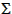i ni

m =i (ni × Mi)

where ni is the number of moles of each constituent, Mi is the molecular weight of each constituent.

Referring back to the last iteration of our combustion equation, we have

CH4 + 2 O20.5186 CO2 + 0.4814 CO + 0.2407 O2 + 1.5051 H2O + 0.2474 H2 + 0.4949 OH

Calculating the molecular weight of the mixture, we have

n = 3.4881 moles,   m = 80.040 g

M = 80.040 / 3.4881 = 22.947 g/mol

Alternatively, if the mole fraction, yi, of each constituent is given, where yi = ni / n, the average molecular weight is found by

M =i (yi × Mi)

Referring to the STANJAN run above, we can see that both the mole fraction and the gas molecular weight is given, where M = 22.519 kg/kmol. This is lower than our derived unit because our example failed to take into consideration all the applicable dissociations.

The constant-pressure specific heat, or heat capacity, Cp, and the constant-volume specific heat, Cv, are useful functions for thermodynamic calculations, particularly for gases, and are defined by the following relationships

Cp = (∂h/∂T)p

Cv = (∂u/∂T)v

where h is specific enthalpy, u is specific internal energy, and T is temperature.

A very important relationship between the constant-pressure and constant-volume specific heats of an ideal gas is found in the equation

Cp – Cv = R'

where Cp and Cv are on a mole basis, and R' is the universal gas constant (8.31446 J/mol-K). This tells us that the difference between the constant-pressure and constant-volume specific heats of an ideal gas is always constant, though both are a function of temperature.

Many thermodynamic solutions use the ratio of the specific heats, k or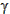, defined as

k = Cp / Cv

Since it is common for thermodynamic references to provide the value of Cp only, from which Cv is calculated, we can combine equations and write

k = 1 / (1 - R' / Cp)

Values of constant-pressure specific heat are typically found by use of an equation, or from tabulated values of Cp as a function of temperature. The Shomate equation is commonly used, which is shown below along with the Shomate coefficients of the products found in our combustion example.

 Gas Phase Heat Capacity (Shomate Equation) Cpo = A + Bt + Ct2 + Dt3 + E/t2 where Cp = heat capacity (J/mol-K), t = Temperature (K) / 1000 Formula Temp. Range A B C D E CO2 1200-6000 58.16639 2.720074 -0.492289 0.038844 -6.447293 CO 1300-6000 35.15070 1.300095 -0.205921 0.013550 -3.282780 H2O 1700-6000 41.96426 8.62253 -1.499780 0.098119 -11.15764 H2 2500-6000 43.413560 -4.293079 1.272428 -0.096876 -20.533862 H 298-6000 20.78603 4.850638x10-10 -1.582916x10-10 1.525102x10-11 3.196347x10-11 O2 2000-6000 20.91111 10.72071 -2.020498 0.146449 9.245722 O 2500-6000 22.18977 -1.318044 0.378308 -0.026069 0 OH 1300-6000 28.74701 4.714489 -0.814725 0.054748 -2.747829 Shomate coefficients obtained from NIST Chemistry WebBook,except monatomic oxygen (O) derived by R.A. Braeunig from data found here.

Calculating the specific heat ratio of a single substance is straightforward. Say we have carbon monoxide at 3,000 K, the specific heat ratio is

From Shomate equation, Cp = 36.8736 J/mol-K

k = 1 / (1 - 8.31446 / 36.8736) = 1.2911

When we have a mixture of gases, we must determine the specific heat of the mixture, where

Cpgas = 1 / n ×i (ni × Cpi)   , or

Cpgas =i (yi × Cpi)

Let's calculate the specific heat ratio for the mixture of gases found in the STANJAN solution to our methane/oxygen problem, noting that the temperature is 3,553.87 K.

Cpgas =i (yi × Cpi) = 49.0786 J/mol-K

k = 1 / (1 - 8.31446 / 49.0786) = 1.2040

Although some computer programs calculate the value of specific heat ratio, STANJAN does not. If STANJAN is used, the user will have to calculate k separately, though this can easily be done through the use of spreadsheet software.

Everything so far has assumed the combustion products consist of a homogenous mixture of gases. When dealing with liquid rocket propellants, this is a fair assumption, as any condensed species (either liquids or solids) will appear in trace quantities only. However, when dealing with solid propellants, a significant portion of the exhaust products will be in a condensed phase, which is evident as visible smoke in the exhaust plume. When the products exist in more than one phase, the mixture is said to be heterogeneous.

A key assumption required is that the condensed-phase particles flow at the same velocity as the gas (i.e. no velocity lag), so the modified equations represent an upper limit on performance. The details are too involved to present here, so only the final outcome is presented, which fortunately, is quite simple. As it turns out, the gas-particle mixture behaves like a gas with a modified specific heat ratio, k. All the fundamental equations remain the same and are fully applicable to two-phase flow, with the only modifications being as follows:

• Molecular weight, M, must take into account the presence of the condensed-phase by calculating the effective molecular weight, which is found by dividing the total system mass, m, by the number of moles of gas in the system, n.

M (effective) = m (total) / n (gas)

• The modified specific heat ratio, also called isentropic exponent, takes two forms, one for conditions where flow velocity (or actually, acceleration) is low, and the other for conditions of flow with high acceleration. Where flow acceleration is low, such as in the combustion chamber,

kmix = 1 / (1 - R' / Cpmix)

where Cpmix is the effective specific heat of the gas and condensed-phase mixture, given by

Cpmix = 1 / n × [i (ni × Cpi) + ns × Cs]

where ni is the number of moles of gas component i, ns the number of moles of condensed-phase component, and n the total number of gas moles.

kmix is the form to use when calculating combustion chamber pressure and characteristic exhaust velocity.

Where flow velocity and acceleration are high, that is, in the nozzle, we have

k2ph = k' × [(1 + Ψ × Cs / Cpgas) / (1 + k' × Ψ × Cs / Cpgas)]

where k' is the specific heat ratio for the gas only mixture; Ψ = ws /(1–ws), where ws is the mass fraction of condensed-phase particles in the exhaust; Cs is the specific heat for the solid (or liquid) mixture in the exhaust; and Cpgas is the specific heat for the gas only mixture. As before,

Cpgas = 1 / ngas ×i (ni × Cpi)

k' = 1 / (1 - R' / Cpgas)

The derivation of this form of modified specific heat ratio assumes a frozen flow condition where no thermal or velocity particle lag is assumed to exist, and is based on the momentum and energy equations for steady isentropic flow.

k2ph is the form to use when calculating exhaust velocity, thrust, thrust coefficient and the other nozzle flow parameters.

Let's now consider an example. Below is a STANJAN run for the combustion of 68% ammonium perchlorate, 18% aluminum, and 14% HTPB at a chamber pressure of 4 MPa.

 ``` Independent relative element system atom population potential Al 6.67111407E-01 -16.5165 Cl 5.78772661E-01 -20.5197 O 2.31509064E+00 -19.0205 H 3.86805792E+00 -9.2472 C 1.03531152E+00 -12.1297 N 5.78772661E-01 -13.7827 Composition at T = 3280.36 K P = 3.948E+01 atmospheres species mol fraction mass fraction mol fraction in the gas in mixture in mixture gas phase Al .15875E-03 .15634E-03 .14634E-03 AlCl .82935E-02 .18897E-01 .76450E-02 AlClO .13303E-02 .38081E-02 .12263E-02 AlCl2 .22637E-02 .80879E-02 .20867E-02 AlCl3 .21732E-03 .10576E-02 .20033E-03 AlO .14196E-03 .22269E-03 .13086E-03 AlOH .42786E-03 .68696E-03 .39441E-03 AlO(OH) .29780E-03 .65205E-03 .27452E-03 CO .27553E+00 .28168E+00 .25398E+00 CO2 .81351E-02 .13067E-01 .74990E-02 Cl .82798E-02 .10713E-01 .76324E-02 H .33101E-01 .12178E-02 .30513E-01 HCl .13549E+00 .18031E+00 .12490E+00 HO .30828E-02 .19136E-02 .28418E-02 H2 .36159E+00 .26606E-01 .33332E+00 H2O .82105E-01 .53989E-01 .75685E-01 NO .18270E-03 .20010E-03 .16842E-03 N2 .79197E-01 .80974E-01 .73005E-01 O .16856E-03 .98432E-04 .15538E-03 condensed species Al2O3(c) .31566E+00 .78192E-01 Mixture properties: gas molal mass = 18.750 kg/kmol T = 3280.363 K P = 4.0000E+06 Pa V = 2.4894E-01 m**3/kg U =-2.8456E+06 J/kg H =-1.8499E+06 J/kg S = 9.6821E+03 J/kg-K Made 3 (T,P) iterations; 11 condensate iterations; 54 gas passes. ```

To perform the calculations, we require the molecular weight and specific heat of each constituent. The following is obtained from the NIST Chemistry WebBook, with Cp valves derived for the chamber temperature of 3280.363 K.

```
Species          M           Cp
g/mol      J/mol-K
---------------------------------
Al            26.982      20.7692
AlCl          62.435      39.0550
AlClO         78.434      62.2791
AlCl2         97.888      58.3019
AlCl3        133.341      83.0688
AlO           42.981      46.9917
AlOH          43.989      60.2511
AlO(OH)       59.988      81.6723
CO            28.010      37.3728
CO2           44.010      62.5638
Cl            35.453      20.9928
H              1.008      20.7860
HCl           36.461      37.5480
HO            17.007      37.1224
H2             2.016      37.6952
H2O           18.015      56.5356
NO            30.006      37.6115
N2            28.013      37.2055
O             15.999      21.0168
Al2O3(c)     101.961     192.4640

```

Referring to the "mol fraction in mixture" in the STANJAN run, we find

ns = 0.078192 mole
n = 1 – 0.078192 = 0.921808 mole (also attainable by summing the gas constituents)

And from "mass fraction in mixture", we find the mass fraction of the condensed species,

ws = 0.31566

Calculating the total mass, we have

m =i (ni × Mi) = 25.256 g/mol

Therefore,

M = 25.256 / 0.921808 = 27.398 g/mol

Further, we findi (ni × Cpi) = 35.6887 J/mol-K

Cs = 192.4640 J/mol-K

Cpgas = 1 / 0.921808 × 35.6887 = 38.7160 J/mol-K

Cpmix = 1 / 0.921808 × (35.6887 + 0.078192 × 192.4640) = 55.0417 J/mol-K

Finally, we calculate the specific heat ratios,

kmix = 1 / (1 – 8.31446 / 55.0417) = 1.1779

k' = 1 / (1 – 8.31446 / 38.7160) = 1.2735

Ψ = 0.31566 / (1 - 0.31566) = 0.46126

k2ph = 1.2735 × [(1 + 0.46126 × 192.4640 / 38.7160) / (1 + 1.2735 × 0.46126 × 192.4640 / 38.7160)] = 1.0698

When to use kmix versus k2ph

Referring to the Rocket Propulsion page, when condensed species are present, the following equations should be solved using kmix: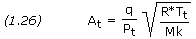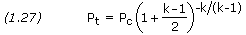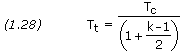And the following should be solved using k2ph: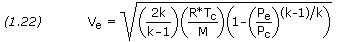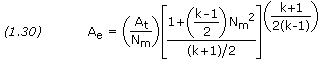Isentropic Compression & Expansion

Here we present two important equations that relate the pressure, temperature, and volume that a gas occupies during reversible compression or expansion. Such a process occurs during the compression and power strokes for an internal combustion engine. The same equations describe the conditions across the compressor and turbine of a gas turbine, or across the nozzle of a rocket engine. The resulting compression and expansion are reversible processes in which the entropy of the system remains constant. Such a process is called an isentropic process.

For isentropic compression and expansion, the effect of pressure on temperature is described by

T2 / T1 = (p2 / p1)1 – 1 / k

and the effect of volume on pressure is

p2 / p1 = (v1 / v2)k

where T1, p1 and v1 are the initial temperature, pressure and volume; T2, p2 and v2 are the final temperature, pressure and volume; and k is the specific heat ratio.

We can also combine equations to find the effect of volume on temperature

T2 / T1 = (v1 / v2)k – 1

Since the expansion of exhaust gases through a rocket nozzle is an isentropic process, the above equations are applicable. Say, for instance, we want to know the temperature at the nozzle exit for a rocket engine having a chamber temperature of 3,250 K, a chamber pressure of 6 MPa, a nozzle exit pressure of 0.07 MPa, and k is known to be 1.23. We have

T2 / 3250 = (0.07 / 6)1 – 1 / 1.23
T2 = 1,414 K

Should you decide to give STANJAN a try, I have some helpful tips learned through my experience using it. This is not instructions on how to use the software.

First, STANJAN comes with a file named TURBINE.DOC that is an example of a STANJAN run for a gas turbine problem. It outlines the steps for finding adiabatic flame temperature and for finding the results of isentropic expansion of the exhaust. Learn to follow this example and you should be able to perform all the calculations described in this article.

Second, STANJAN comes with a limited number of species data files, so you're certainly going to have to create some of your own if you plan to get serious about using the software. Included with STANJAN is a file named SPECIES.DOC that explains how the data files are formatted. Below is an excerpt showing a sample data entry for carbon dioxide.

 ``` Species name (8 chars) Atomic composition: Left justified in Col 1 | number right justified in | Date of data the field followed by | 8 chars starting Col 10 atom symbols (2 chars) | | left justified in the | | Cols 19-28 field (upper or lower | | mol. wt, g/mol case, but you must use | | | the same characters for a | | | cols 29-38 given atom throughout | | | enth. form the data file). Must be | | | at 298.15K, positioned as below; | | | kcal/mol 6(I3,A2) starting in | | | | col 49. | | | | Cols 39-48 . | | | | 0.0 for gas; . | | | | density, g/cc . | | | | for liquid or . | | | | solid ............................... | | | | | | | | | | | | | | | | | .. | .. | .. | .. | .. | .. CO2 9/30/65 44.00995 -94.054 0.000 1C 2O 0 0 0 0 47.769 51.072 51.127 53.830 56.122 58.126 59.910 61.522 62.992 64.344 65.594 66.756 67.841 68.859 69.817 70.722 71.578 72.391 73.165 73.903 74.608 75.284 75.931 76.554 77.153 77.730 78.286 78.824 79.344 79.848 80.336 80.810 81.270 81.717 82.151 82.574 82.986 83.388 83.780 84.162 84.536 84.901 85.258 85.607 85.949 86.284 86.611 86.933 87.248 87.557 87.860 88.158 88.451 88.738 89.021 89.299 89.572 89.841 90.106 90.367 -0.816 0.000 0.016 0.958 1.987 3.087 4.245 5.453 6.702 7.984 9.296 10.632 11.988 13.362 14.750 16.152 17.565 18.987 20.418 21.857 23.303 24.755 26.212 27.674 29.141 30.613 32.088 33.567 35.049 36.535 38.024 39.515 41.010 42.507 44.006 45.508 47.012 48.518 50.027 51.538 53.051 54.566 56.082 57.601 59.122 60.644 62.169 63.695 65.223 66.753 68.285 69.819 71.355 72.893 74.433 75.976 77.521 79.068 80.617 82.168 Lines 2-7 are the values of S(T,1 atm), cal/mol-K, as read from the JANNAF tables at 200, 298.15,300,400,500,....,6000 K. Lines 8-13 are the values of H-H(298.15), kcal/mol, as read from the JANNAF tables at 200, 298.15,300,400,500,....,6000 K. ```

STANJAN already includes the gaseous species you'll commonly encounter in most combustion reactions, that is, those containing C, H, N and O. This will suffice for calculations involving most liquid propellants. However, if you plan to perform calculations involving solid propellants, new elements will be introduced into the exhaust, such as Al and Cl. This will necessitate the creation of an entirely new group of species data files.

Data files can be easily edited and/or created in a text editor such as Notepad or WordPad. The values that populate the data files can be found and/or calculated using NIST Chemistry WebBook or, even easier, using NIST-JANAF Thermochemical Tables. Be advised, however, that the STANJAN data files use units of calories instead of Joules. The NIST Chemistry WebBook allows the selection of either calorie-based or Joule-based data. The NIST-JANAF Thermochemical Tables site, although presenting the data in a more convenient format, must be converted from Joules to calories, where 1 calorie = 4.184 Joules.

You will most definitely need to create you own data files for the reactants, as STANJAN doesn't include any of the common rocket propellants. However, there's a short cut that greatly simplifies this task. If you always assume that your reactants are at an initial temperature of 298.15 K, i.e. the base temperature, there is no need to populate the data files with all the S and H-H(298.15) data, as this is used only when the temperature varies from 298.15 K. You need to complete the data on the first line only, making your reactant data files look like the following example for methyl hydrazine:

 ``` CH6N2 1951 46.07170 12.940 0.866 1C 6H 2N 0 0 0 .000 .000 .000 .000 .000 .000 .000 .000 .000 .000 .000 .000 .000 .000 .000 .000 .000 .000 .000 .000 .000 .000 .000 .000 .000 .000 .000 .000 .000 .000 .000 .000 .000 .000 .000 .000 .000 .000 .000 .000 .000 .000 .000 .000 .000 .000 .000 .000 .000 .000 .000 .000 .000 .000 .000 .000 .000 .000 .000 .000 .000 .000 .000 .000 .000 .000 .000 .000 .000 .000 .000 .000 .000 .000 .000 .000 .000 .000 .000 .000 .000 .000 .000 .000 .000 .000 .000 .000 .000 .000 .000 .000 .000 .000 .000 .000 .000 .000 .000 .000 .000 .000 .000 .000 .000 .000 .000 .000 .000 .000 .000 .000 .000 .000 .000 .000 .000 .000 .000 .000 ```

Of course, assuming a temperature of 298.15 K appears, at first, to be a problem when using cryogenic propellants, but there's a way around this. Besides, STANJAN allows a minimum starting temperature of 200 K, so we can't set our reactants to cryogenic temperatures even if we wanted to. Furthermore, STANJAN doesn't allow reactants of different temperatures, so it can't handle a scenario like RP-1 and LOX, where the temperatures are vastly different.

The solution is a simple trick; in the field where you would normally enter the enthalpy of formation, replace this with the enthalpy of the cryogenic liquid at its boiling point. I earlier demonstrated this when calculating the enthalpy of LOX to be -12.88 kJ/mol, or -3.078 kcal/mol. Now when entering a temperature of 298.15 K, you'll actually get the enthalpy of the cryogenic liquid.

Finally, I like to create separate data files for the reactants and the products. And for the products I like to create several data files, each one representing a different group of elements. For example, I have data files HO.DAT, CHO.DAT, CHNO.DAT, etc. Thus, if my reaction contains only the elements C, H and O, I'll select the file CHO.DAT, which contains all the constituents normal to such a reaction – C, CO, CO2, H, H2, HO, H2O, O and O2. This reduces the number of keystrokes require versus having to select the applicable constituents from an all-inclusive list of products.

STANJAN will operate on a PC running Windows XP or earlier. It will also work with Windows 7 Professional in XP Mode. Whether or not it will run on other platforms is unknown.

Compiled, edited and written in part by Robert A. Braeunig, 2012.
Bibliography